# Quadrangle

(diff) ← Older revision | Latest revision (diff) | Newer revision → (diff)
Jump to: navigation, search

In elementary geometry a quadrangle is a figure consisting of four segments intersecting in four (corner) points.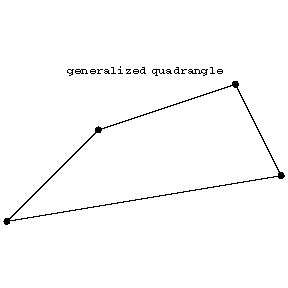Figure: q076000a

Note that each point is incident with 2 lines, each line is incident with 2 points, that there is at most one line passing through two distinct points, that two lines intersect in at most one point, and that for a point and a line not incident with that point there is a unique line through that point intersecting the given line.

These properties exemplify the simplest case of a generalized quadrangle. This is an incidence system, i.e. a (symmetric) incidence relation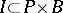between points (the set) and lines (or blocks, the set) such that

i) for each pointand linenot passing throughthere is precisely one pairwithon,onand.

A generalized quadrangle can be seen as a very special kind of bipartite graph (cf. Graph, bipartite), obtained by taking as its vertex set the disjoint union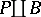and with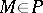,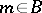connected if and only ifis on.

Interchangingandone obtains the dual generalized quadrangle.

A generalized quadrangle is non-degenerate if there is no point that is collinear with all others, where two points are collinear if they are on a common line.

A finite generalized quadrangle of order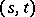is one that satisfies i) above and also

ii) each point is incident with precisely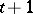lines and there is at most one line through two distinct points;

iii) each line haspoints and two lines intersect in at most one point.

A simple example of a finite generalized quadrangle of order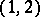is depicted belowFigure: q076000b

This is also an example of a grid, which is an incidence structure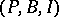with,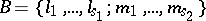with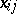on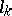if and only if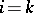andonif and only if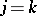.

There are three known families of generalized quadrangles associated with the classical groups; these are known as classical generalized quadrangles. There are also others, for instance coming from ovoids (cf. Ovoid).

Generalized quadrangles were introduced by J. Tits [a1] and he also described the classical ones and the first non-classical ones. More generally one considers generalized-gons, [a4].

How to Cite This Entry:
Quadrangle. Encyclopedia of Mathematics. URL: http://encyclopediaofmath.org/index.php?title=Quadrangle&oldid=13574